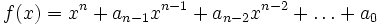Ring over which every monic polynomial has finitely many roots

This article defines a property of commutative unital rings; a property that can be evaluated for a commutative unital ring
View all properties of commutative unital rings
VIEW RELATED: Commutative unital ring property implications | Commutative unital ring property non-implications |Commutative unital ring metaproperty satisfactions | Commutative unital ring metaproperty dissatisfactions | Commutative unital ring property satisfactions | Commutative unital ring property dissatisfactions

Definition

Let$R$ be a commutative unital ring. We say that$R$ is a ring over which every monic polynomial has finitely many roots if every monic polynomial over$R$ has finitely many roots, i.e., for any monic polynomial:$f(x) = x^n + a_{n-1}x^{n-1} + a_{n-2}x^{n-2} + \dots + a_0$,

the set:$\{ a \in R \mid f(a) = 0\}$

is finite.